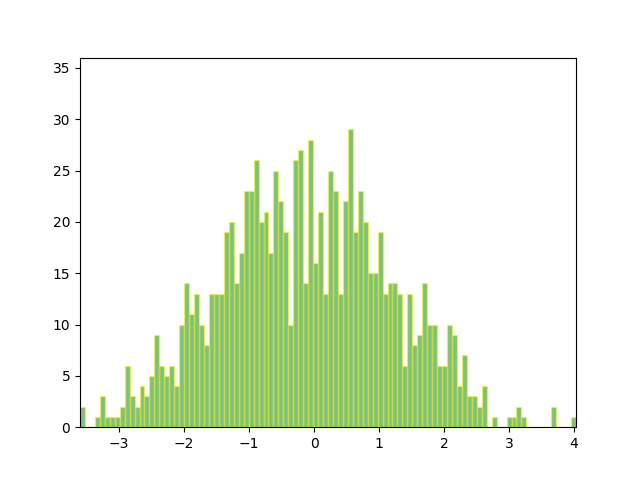# Animated histogram¶

Use a path patch to draw a bunch of rectangles for an animated histogram.

import numpy as np

import matplotlib.pyplot as plt
import matplotlib.patches as patches
import matplotlib.path as path
import matplotlib.animation as animation

# Fixing random state for reproducibility
np.random.seed(19680801)

# histogram our data with numpy
data = np.random.randn(1000)
n, bins = np.histogram(data, 100)

# get the corners of the rectangles for the histogram
left = np.array(bins[:-1])
right = np.array(bins[1:])
bottom = np.zeros(len(left))
top = bottom + n
nrects = len(left)


Here comes the tricky part -- we have to set up the vertex and path codes arrays using plt.Path.MOVETO, plt.Path.LINETO and plt.Path.CLOSEPOLY for each rect.

• We need 1 MOVETO per rectangle, which sets the initial point.
• We need 3 LINETO's, which tell Matplotlib to draw lines from vertex 1 to vertex 2, v2 to v3, and v3 to v4.
• We then need one CLOSEPOLY which tells Matplotlib to draw a line from the v4 to our initial vertex (the MOVETO vertex), in order to close the polygon.

Note

The vertex for CLOSEPOLY is ignored, but we still need a placeholder in the verts array to keep the codes aligned with the vertices.

nverts = nrects * (1 + 3 + 1)
verts = np.zeros((nverts, 2))
codes = np.ones(nverts, int) * path.Path.LINETO
codes[0::5] = path.Path.MOVETO
codes[4::5] = path.Path.CLOSEPOLY
verts[0::5, 0] = left
verts[0::5, 1] = bottom
verts[1::5, 0] = left
verts[1::5, 1] = top
verts[2::5, 0] = right
verts[2::5, 1] = top
verts[3::5, 0] = right
verts[3::5, 1] = bottom


To animate the histogram, we need an animate function, which generates a random set of numbers and updates the locations of the vertices for the histogram (in this case, only the heights of each rectangle). patch will eventually be a Patch object.

patch = None

def animate(i):
# simulate new data coming in
data = np.random.randn(1000)
n, bins = np.histogram(data, 100)
top = bottom + n
verts[1::5, 1] = top
verts[2::5, 1] = top
return [patch, ]


And now we build the Path and Patch instances for the histogram using our vertices and codes. We add the patch to the Axes instance, and setup the FuncAnimation with our animate function.

fig, ax = plt.subplots()
barpath = path.Path(verts, codes)
patch = patches.PathPatch(
barpath, facecolor='green', edgecolor='yellow', alpha=0.5)